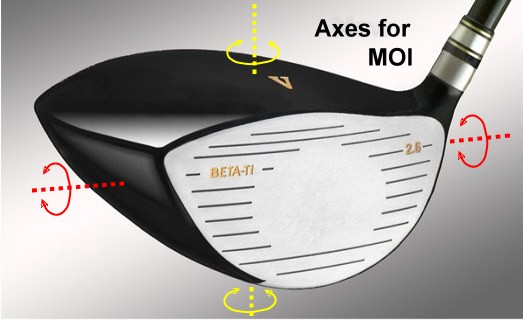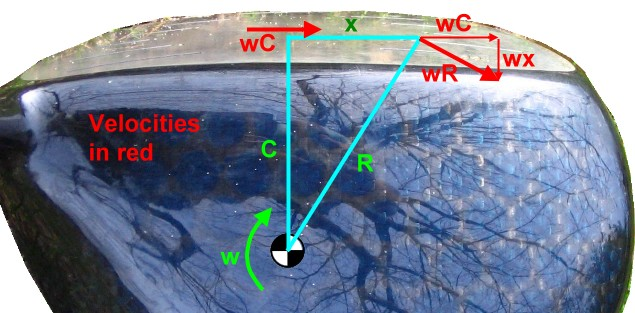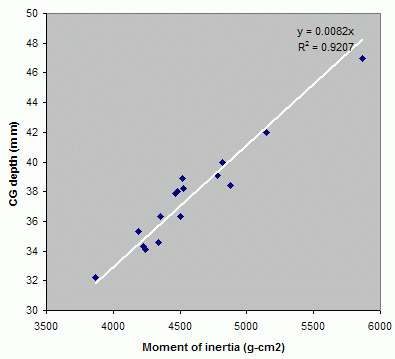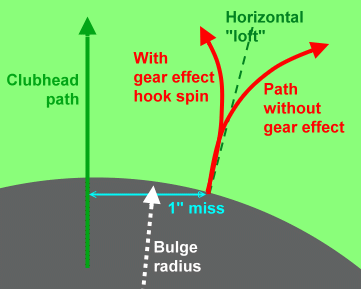# All about Gear Effect - 1

Dave Tutelman  --  February 19, 2009

## Horizontal gear effect

Gear effect is caused by the rotation of the clubhead around its center of gravity, when a ball is struck off-center. Let's look at that a little more closely.Figure 1-1

When the ball is struck by the clubhead, each exert force on the other. The force of the ball on the clubhead either goes through the center of gravity (hereinafter called the CG) or not. If the force is not through the CG, then the CG tends to stay were it is, so the force generates a torque that wants to rotate the clubhead around its CG.

In the picture, the force misses passing through the CG by a distance x. So the torque wanting to turn the clubhead is the force times x.

Also in the picture is C, the distance the CG is back from the face. That will figure in our analysis.

If the ball misses to the right or left of the CG, the clubhead will rotate right or left, producing hook spin or slice spin. This is what is usually meant by gear effect, but is more properly called horizontal gear effect because the head is rotating in a horizontal plane.

By the same token, a miss above or below the CG will produce topspin or backspin. (Well, it probably won't produce enough topspin to overpower the backspin that's there due to the loft of the clubhead. So, strictly speaking, it is reducing backspin, not producing topspin.) This is called vertical gear effect, because the spin is in a vertical plane.Figure 1-2
(The diagram of the driver head is adapted from a photo
of the Vector 460-TS driver from The Golf Coast
)

Since all gear effect is based on the clubhead rotating due to impact, we will need to know the moment of inertia of the clubhead. Actually, we will need to know two moments of inertia. MOI is not a property of an object, but of an object and an axis of rotation. For horizontal gear effect, the clubhead rotates about the yellow axis in the diagram, which goes through the CG. For vertical gear effect it is the red axis, which also goes through the CG.

## Horizontal gear effect

Let's get on with the business of coming up with a formula for the spin due to gear effect. Here are the symbols we will use and what they represent.
 Vb Initial velocity of the ball. m Mass of the ball. Ih Moment of inertia of the clubhead about the yellow axis in Figure 1-2 (i.e.- for horizontal gear effect). We use the engineer's notation of I rather than the golf jargon MOI. Iv Moment of inertia of the clubhead about the red axis in Figure 1-2 (i.e.- for vertical gear effect). C Distance from clubface to CG (see Figure 1-1). x Distance from force to CG (see Figure 1-1). F(t) The force between clubface and ball. This varies during the time of impact, and is zero at all other times. ω Angular velocity of the clubhead as the ball leaves the clubface. v Sideways velocity of the clubface as the ball separates from it. This sideways velocity is what imparts gear effect spin. s Initial spin of the ball due to gear effect, in revolutions per minute (rpm).
We will use standard (mks - meter/kilogram/second) units to keep the math simple. At the end, we will convert the formula to work in more familiar units.

Figure 1-1 shows the clubface striking the ball a distance x from the CG. There is a force F(t) exerted by the ball on the clubhead, and of course an equal and opposite F(t) exerted by the clubhead on the ball. The ball is accelerated during the half millisecond of impact to velocity Vb, which gives it a momentum of mVb. Because momentum equals impulse:
mVb  =  F(t)dt
The impulse (the right-hand side of the equation) links the ball to the clubhead. Exactly the same impulse is imparted to the clubhead at the point of contact. We can express the impulse on the clubhead as a torque impulse about the CG, simply by multiplying the force function by the lever arm x. So now we have the making of the rotational impulse-momentum equation:
Ihω  =  xF(t)dt
Since F(t) and thus F(t)dt is the same for both equations, we can write it as:
Ihω  =  xmVb
Which is easily solved for the rotational velocity as:
 ω  = xmVb Ih
That was remarkably easy. Yes, some integral calculus showed up at one point; but everything there canceled out before we had to worry about how to do the integration.

And it was remarkably powerful. Now we have the rotational motion of the clubhead as the ball is departing. It's actually pretty easy to get the spin from this. No more physics, just geometry.Figure 1-3

In Figure 1-3, the clubhead is rotating about its CG at ω radians per second. (It's "w" in the diagram because of the limitations of my drawing program.) Since the linear velocity tangent to the rotation is the radius times ω, the sideways velocity at the middle of the clubface is ωC.

The velocities at the point of impact -- a distance x from the middle of the clubface -- is only a little harder. This time the radius is R, and the velocity ωR is again perpendicular to the radius, but this time at an angle to the clubface. We could calculate R using either the Pythagorean Theorem or trigonometry (I did it both ways the first time through). But it won't matter. Because the triangle of velocity vectors is a right triangle similar to the triangle of distances, we can just write the two velocities down by inspection as ωC and ωx. (If you do all the calculations, what you got for R will cancel out.) So we have:
 ωC The sideways motion of the face that is synchronized to the rotation of the ball as the ball leaves the face. Earlier, we called that v in our notation. Obviously this is how we are going to compute the spin. It's all easy from here. ωx The backwards motion of the face away from the ball. This is why the ball does not have as much speed on an off-center hit. We could compute the speed loss from this, but we have other fish to fry here.
To go from  v = ωC to spin in rpm requires:
• Dividing by the circumference of a golf ball. The velocity v is in meters per second. Divide by the circumference in meters and we have revolutions per second.
• Multiplying by 60 to go from revolutions per second to revolutions per minute.
s   =
 v 60 .043π
=   444 v
= 444 ωC
=
 444 Vb C x m Ih
Since the golf ball's mass is within a fraction of a gram of 46g (or 0.046Kg):
 s  =  20.4 Vb C x Ih (Equation 1)
This is the result we were after! And it is remarkably simple.

## Real numbers, and a sanity test

When we get to talking about real numbers, it would be more convenient if we could use the units we normally use as clubmakers. (I live in the USA. Apologies to those of you reading this who are already metric.)
• Vb in miles per hour
• C and x in inches.
• Ih in g-cm2.
Let's crank the unit conversion factors into the formula.
s
 20.4 * .447 Vb .0254 C * .0254 x 10-7 Ih
 58,830 Vb C x Ih
(Equation 2)
While we're at it, the average force on the clubhead during impact, using units of miles per hour and pounds of force, is:
F  =  9.24 Vb
We may need that from time to time.

### Numbers for real drivers

Before we proceed any further, we need to know Ih and C for real drivers. (We will also need bulge radius, if we want to consider the actual trajectory implications.)

First the clubhead's horizontal moment of inertia Ih. I started by looking in component catalogs for listings of Ih. The only catalog I could find that gives this information is Golfsmith's online web site. Their modern 460cc drivers covered a range from 4400 to 5800 g-cm2. The "typical" new driver seemed to be about 5200 g-cm2. I used that value in the first draft of this study.

Jeff Summitt challenged this, and pointed me to a Japanese web site that reprinted tests of OEM clubs from Alba magazine. It had actual measured values for 17 current driver models. (I have duplicated their data in tabular form for ease of viewing.) These showed a much larger range of MOI than the Golfsmith catalog, and generally lower values. Only two were over 5000 g-cm2, and there was one under 4000. The average was under 4600 g-cm2, well below the 5900g-cm2 limit imposed by the Rules of golf.

Conclusion: Even allowing for the fact that almost every driver on the market today is at the 460cc volume limit in the Rules, very few so far are pushing the Ih limit of 5900 g-cm2. It takes conscious allocation of peripheral weight, plus broadening and lengthening the head, to approach the legal limit.

Next, we want to find the distance C from the CG to the face. This is generally referred to as the "CG depth". Again, I started with resources on hand. I measured the CG depth of four fairly recent driver heads: Vector 460TS, Wishon 915CFE, KZG Gemini, and Dynacraft Prophet. They varied, but not much; I found a range of 1.2" to 1.3", probably no bigger a range than the imprecision of my measurements.

Then I recognized that the Alba test data from the Inpakuto.com web site contained not only MOI data but CG depth as well. When I checked it out, I saw a variation from 32mm to 47mm; in inches, that's 1.25" to 1.85". Once again, we see a much larger range than I was led to believe by easily available information. This data presents two problems for me:
1. My measurements (1.2"-1.3") are hanging off the low end of the range (1.25"-1.85").
2. It looks like there will be a wide range of gear effect spin from different drivers, which we have to take into account.
A look at the way CG depth was measured by Alba can explain #1. They measured from the leading edge of the driver back to the CG. But the leading edge is the bottom of the face. Because of loft, the middle of the face will be closer to the CG, and the middle of the face is what I measured. At driver lofts, the Alba measurements will be about 14% greater than C, which is measured from the middle of the face. If this additional 14% is taken into account, my measurements are more like 1.4"-1.5", well within the range of the tested drivers.

Problem #2, the large variation in C, is more difficult.

But wait! Eyeballing the data suggests that larger MOI goes hand in hand with larger CG depth. I went through a few statistical tests to see whether that is a dependable relationship:

 MOI CG depth Ratio I/C Average 4575 37.7 121 Standard Deviation 462 3.55 3.18 Std Dev as percent of average 10% 9.4% 2.5%
• First I determined the average and the standard deviation of the moment of inertia, the CG depth, and the ratio of the two. (Actually, I first removed one "wild data point", the Callaway Legacy driver, which was way off the statistical trend of the other heads.) The result (shown in the table): while MOI and CG depth both had a lot of variation -- a standard deviation of about 10% -- their ratio only had a standard deviation of 2.5%. That suggests a very strong correlation.Figure 1-4
• Next I used Excel's charting function to do a scatter plot of CG depth vs MOI. Figure 1-4 shows the points lining up very visibly. Excel supports adding a trendline that best fits the data; that is the white line in the graph. R2 is a measure of correlation, with 1.0 being perfect correlation. For this data, it is .92, which is very strong.

Why is that important? Turns out it is very important. Remember that our equation for spin has C in the numberator and Ih in the denominator. So, if the ratio of C to Ih is constant, then the spin does not vary much with CG depth or moment of inertia -- because they track one another and balance out.

So we are going to pretend that there is only one driver in existence, one that lives on the white line in Figure 1-4. It can be anywhere on the white line; all points on the line will give equal spin. Let's just pick a point on the line, and see what happens to eqaution 2. Let's try Ih=5100 and CG depth=42mm. C is 14% less than CG depth, so C=36mm=1.42inches.

We can fold this back into Equation 2 and get:
s
 58,830 Vb C x Ih
 =  58,830 Vb 1.42 x 5100
= 16.4 Vb x (Equation 2a)
This is amazingly simple, and is a good approximation for a wide range of drivers. If we use this to estimate gear effect spin, the statistical error will be only 2.5%; that's only 25cpm out of 1000cpm of gear effect.

Finally, we will need bulge radius. Drivers use bulge to compensate for gear effect. Any full-trajectory implication of gear effect must be calculated with bulge figured in.

The range of bulge that I found in catalogs that list bulge was 10"-13" radius of curvature. The most common number was 12", with 10" in second place. We will use a typical 12" bulge for the rest of our calculations.

### Sanity testFigure 1-5

Does equation 2a give correct results? We have a way to check this. The face bulge on a driver is used to counter gear effect; it starts a miss to the right of center (like the example in our diagrams) out to the right so the hook spin brings it back towards the center.

Figure 1-5 is a top view of a driver head with an exaggerated bulge curvature. The hit depicted is a miss 1" toward the toe. Because of curvature, the ball is struck as if there were a "loft" in the horizontal as well as the vertical direction. Without any gear effect, the shot will start right (because of the bulge) and slice even more to the right (because of the horizontal "loft"). But gear effect contributes hook spin, with the intent of getting the ball back on-line.

Let's assume that the designers of the driver know what they are doing. If so, the combination of bulge curvature and gear effect should result in a much smaller error in the actual drive than the gear effect alone with no bulge curvature. So let's test our formula against our typical driver. We will use equation 2a and a 12" bulge radius.

The table below shows how gear effect and bulge counter one another. The numbers come from:
• Simple trigonometry; e.g. - the "loft" is the arctan of (x divided by the bulge radius).
• Equation 2a above.
• TrajectoWare Drive software.
With our ball speed of 150mph, the golfer will hit a typical well-suited (11°) driver about 240 yards (assuming zero angle of attack, center impact, etc). So we will look at the side error using an 11° driver. As noted above, we assume a 12" bulge. We use C=1.3" where we need C -- roughly the average value from the Alba tests.

 Miss (how far towards toe) Horizontal "loft" due to bulge Horizontal "launch angle" Slice spin due to bulge Hook spin due to gear effect Hook spin (net) Total side error Hook without bulge ¼ 1.2° 1.1° 338 553 215 3 yards left 20 yards left ½" 2.4° 2.1° 676 1113 437 6 yards left 38 yards left 1" 4.8° 4.2° 1354 2192 838 10 yards left 61 yards left 1½" 7.1° 6.2° 2005 3294 1289 11 yards left 72 yards left

The table shows the steps to computing the total side error -- the green column. (For more detail on how the computation was done, use this link.) Compare the green column with the red column, which is the error which is what the error would have with a flat face, no bulge.

That is a pretty good confirmation of both the formula and the design of the driver. For each miss -- even missing the "sweet spot" by an inch and a half, almost off the face -- the total side error is at least six times less than it would have been without bulge. The errors due to bulge do a fine job of canceling out the errors due to gear effect. And they could be even better. A few calculations at a 10½" bulge radius shows very small errors, in the vicinity of a yard.

This data strongly suggests that the formula for spin is sound.

Interesting historical note:
In 1946, a patent was issued to John Baymiller and Robert Vose of Spalding Golf. It disclosed a formula relating the optimum bulge radius to the CG depth. The formula is largely empirical, based on many measurements of real hits with the wooden clubs then current. For C=1.3", the formula recommends an optimum bulge of 9½", just an inch less than our analysis-based optimum seems to be. Bearing in mind that the moment of inertia of a clubhead was lower in 1946, you would expect the ideal C to be smaller.
Interesting personal note: John Baymiller is the father of Bernie Baymiller, a custom clubmaker and a friend of mine. He gave me a copy of the patent long ago, but I finally understand it better now.Last modified - Feb 19, 2009